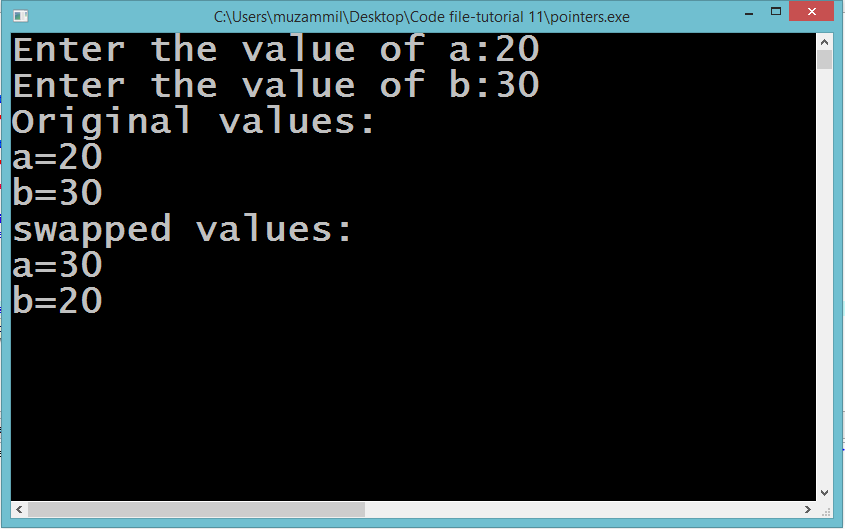# Introduction to Pointers

## Introduction to Pointers

In this part you will learn: 1. C syntax 2. Pointers 3. Pointers declaration 4. Showing output

### What is a Pointer?

Whenever we declare variable our computer stores it in at a memory location in the memory. A unique address is assigned to every variable made by the user either its on run time or compile time. We use pointers to access those address whenever we want to and use the value that is stored on that particular address. Pointer is a special kind of variable. Pointers are designed to store the memory address i.e. the address of another variable. Declaring a pointer is same as declaring any variable the difference is that between the type of variable and the name of the variable we place a asterisk ‘*’. The asterisk can be written either with the type or the name of the variable. Like this. int* p or int *p (they both are the same). When we write this statement this means that we declared a integer type pointer that will point to an integer type variable. In C, especially when we are talking about pointer we call this symbol as dereference operator. Because when write this operator with any pointer that means that we are accessing the value of the address that is stored in that certain pointer. When studying pointer you should also know about another symbol called ampersand ‘&’. You would have seen and used this symbol when you write the scanf() function. This symbol is used to access the address of any variable. Swapping With Pointers Open Dev C++ then File > new > source file and start writing the code below.
`#include<stdio.h>#include<conio.h>`
These two are the most common and basic header files. The first one stdio.h is used to grant us most of the basic functions of C like Input and Output functions. The second one conio.h provides us functions like getch() which is used to pause our screen until we press a button.
`nt main(){  	int a,b; 	printf("Enter the value of a:");	scanf("%d",&a); 	printf("Enter the value of b:");	scanf("%d",&b); 	int *p=&a,*q=&b; 	printf("Original values:");	printf("\na=%d\nb=%d",*p,*q);`
Now in this program we are swapping the values of two integers that were entered by the user. We are doing this with the help of variables. Firstly we declared two variables of integer type and took input from the user. We stored the input in a and b respectively. Then we declared the two pointers of integer type and gave them the address of a and b respectively. We did this using the ampersand sign. This assigns the address of the memory location where the value of a and b are stored to the pointers. Then we print the value of a and b through p and q by using the dereference operator. The meaning of writing asterisk sign with pointer name is value at that address.
`	p=&b;	q=&a; 	printf("\nswapped values:");	printf("\na=%d\nb=%d",*p,*q);  	getch();`
This is the part where we swap the value of a and b. We did this by assigning the pointers p and q opposite addresses. Means that first we assigned pointer p the address of ‘a’ and q the address of ‘b’. Now we assigned p the address of ‘b’ and q the address of ‘a’. Now our pointer p is pointing at the value that was stored in ‘b’ and q is pointing to a value that was stored in ‘a’ At last when we printed the value using dereference operator we got the swapped values of ‘a’ and ‘b’. Execute > compile then Execute > run Output